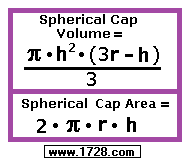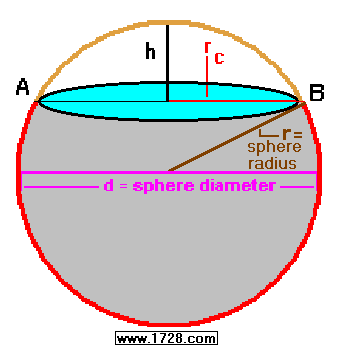Spherical Cap Calculator For a simpler partially-filled sphere calculator, click here. For a sphere volume calculator, click here. It calculates volume when you input radius, diameter, circumference, area or volume.Click on the 2 variables you know:       Sphere Radius 'r' & Chord 'AB'       Sphere Radius 'r' & Cap Height 'h'       Chord 'AB' & Cap Height 'h'       Chord 'AB' & Arc 'AB'             Click here to see the formulas for a partially-filled sphere. Significant Figures >>> Numbers are displayed in scientific notation in the amount of significant figures you specify. For easier readability, numbers between 1,000 and -1,000 will not be in scientific notation but will still have the same precision. You may change the number of significant figures displayed by changing the number in the box above. Most browsers, will display the answers properly but if you are seeing no answers at all, enter a zero in the box above, which will eliminate all formatting but at least you will see the answers. RETURN TO GEOMETRY INDEX Return To Home Page Copyright © 1999 -     1728 Software Systems